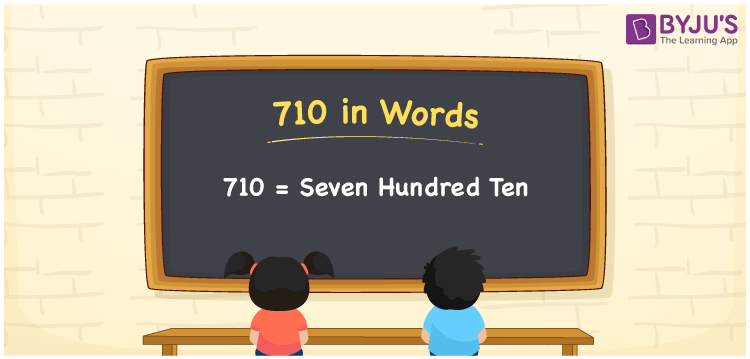# 710 in Words

710 in words can be written as Seven Hundred Ten. This article will help you to understand the concept of counting which is a crucial concept in Mathematics. If you buy a fan for Rs. 710, then you can say that “I bought a fan for Seven Hundred Ten Rupees”. Memorize the place value concept well from the reference materials available at BYJU’S. So, the number 710 can be read as “Seven Hundred Ten” in words.

 710 in words Seven Hundred Ten Seven Hundred Ten in Numbers 710

## 710 in English Words## How to Write 710 in Words?

Learn the exact method how numbers can be written in words. Refer to the chart and convert numbers in words with ease.

 Hundreds Tens Ones 7 1 0

710 in expanded form can be written as:

7 × Hundred + 1 × Ten + 0 × One

= 7 x 100 + 1 x 10 + 0 x 1

= 700 + 10

= 710

= Seven Hundred Ten

Hence, 710 in words is written as Seven Hundred Ten.

710 is a natural number that precedes 711 and succeeds 709.

710 in words – Seven Hundred Ten

Is 710 an odd number? – No

Is 710 an even number? – Yes

Is 710 a perfect square number? – No

Is 710 a perfect cube number? – No

Is 710 a prime number? – No

Is 710 a composite number? – Yes

## Frequently Asked Questions on 710 in Words

Q1

### How to write 710 in words?

710 can be written in words as “Seven Hundred Ten”.
Q2

### Write Seven Hundred Ten in numbers.

Seven Hundred Ten in numbers can be written as 710.
Q3

### Is 710 an even number?

710 is an even number as it is completely divisible by 2.